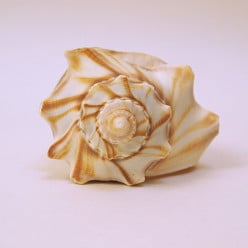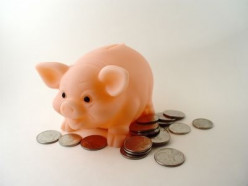Teaching Math

•8

Math Workshop Model: Teaching Elementary Mathematics

7 years ago

The math workshop model is an interactive method for teaching mathematics. The structure, ideas to get started, and how math workshop impacts students are included.

•9

Converting within the Metric System using the Metric Staircase

7 years ago

How to convert in the Metric system using the Metric Staircase as a visual example to help you go from mili all the way to Kilo.

•0

What’s Singapore Math? - Why Singapore’s Children are so Good at Math!

4 months ago

I was worried about the performance of my children in mathematics over other required subjects. However, after, I learn about Singapore math program. They started solving problems with ease.

•7

How To Convert From Cm To Inches (And Back)

7 years ago

Learn how to convert from cm to inches and backward from inches to cm. This conversion is quite simple and easy to comprehend. I will show you basic formulas for better understanding of relation between measurement units. In addition you can find here practical examples for using formulas and many...

•4

Pi & the Circumference of a Circle, Easy Project for Visual Reinforcement.

20 months ago

A easy activity for pi day or any day to talk about circumference of a circle. This is an easy project to relate the circumference of a circle and pi

•28

Football, Absolute Value and A Life Size Number Line Too: A Middle School Math Hands-On Lesson

7 years ago

Needing a fun way to teach absolute value to your middle schooler? Take a look at this lesson plan using football and a life size number line!

•23

More Hands-On Math--Using the Pythagorean theorem

7 years ago

This Hub is about teaching Middle School Math through the use of a hands-on activity. The topic being taught here is the pythagorean theorem and the hands-on activities are the Superbowl (buying an HDTV and TV stand) and the Baseball World Series (the baseball diamond).

•24

Extra Credit: Math Cartoon--"Cathy" and How much does stuff cost?

20 months ago

This Hub is about teaching Middle School Math through the use of a hands-on extra credit cartoon activity. The topic being taught here is budgeting and the hands-on activity is using the cartoon of Cathy from an NCTM article series.

•24

Understanding a Billion

4 years ago

Activities to help children understand larger numbers. How can we comprehend numbers in the millions, billions and beyond? Fun, hands-on lessons in place value and quantities in the billions...

•2

Fraction Help. A complete guide to everything you need to know about fractions in your math exam.

7 years ago

Here you will learn all the basics of fractions. You will find help on converting between improper and mixed numbers, adding fractions, subtracting fractions, multiplying fractions, dividing fractions, equivalent fractions and simplifying fractions.

working